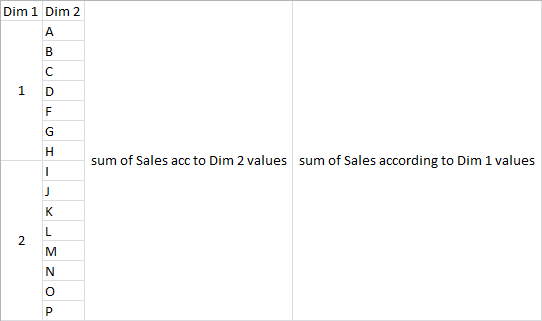# QlikView App Dev

Discussion Board for collaboration related to QlikView App Development.

Announcements
On May 18th at 10AM EDT we will answer your QlikView questions live. REGISTER
cancel
Showing results for
Did you mean:Not applicable

## Effect of restricting dimension on totals in expressions

Does restricting the dimensions affects sum ( total ...) in expressions?

I am restricting my dimensions on rank (aggregate function) and then calculating total in expressions.

I am not getting true values.

Any ideas from anyone would be appreciated.

2 RepliesMVP

Yes it does. Expressions calculate results based on the values of the dimensions shown in your chart. Perhaps you can use a variable or set analysis expressions. Can you post an example document that explains what you're trying to do?

talk is cheap, supply exceeds demandNot applicable
Author

Here, Dim 1 is my calculated dimension (restricted by rank).

i want to see sum of sales in first expressions according to Dim 2 values e.g. sum of sales of A, B, C and so on in respective rows and in second expression, i want to see sum of sales according to Dim 1 values i.e. sum of sales of 1, 2 etc respectively. In second expression i want result irrespective of Dim 2 values.i am getting issues while calculating expression 2.